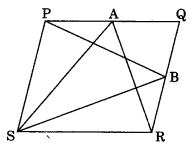CBSE Class 9 Areas of Parallelograms and Triangles MCQ New

## CBSE Class 9 Areas of Parallelograms and Triangles MCQ By Clarify Knowledge

CBSE Class 9 Areas of Parallelograms and Triangles MCQ New Pattern 2022

## CBSE Class 9 Areas of Parallelograms and Triangles MCQ Table

Question 1.
Two parallelograms are on equal bases and between the same parallels. The ratio of their areas is
(a) 1 : 2
(b) 1 : 1
(c) 2 : 1
(d) 3 : 1

Question 2.
ABCD is a quadrilateral whose diagonal AC divides it in two parts of equal area, then ABCD is a
(a) rectangle
(b) rhombus
(c) parallelogram
(d) need not be any of (a), (b) or (c)Answer

Question 3.
If a triangle and a parallelogram are on the same base and between same parallels, then the ratio of the area of the triangle to the area of parallelogram is
(a) 1 : 3
(b) 1 : 2
(c) 3 : 1

Question 4.
The median of a triangle divides it into two
(a) isosceles triangle
(b) congruent triangles
(c) right angled triangle

Question 5.
PQRS is a parallelogram and A and B are any points on PQ and QR. If ar(PQRS) = 48 cm², then ar(ΔPBS) + ar(ΔASR) is equal to(a) 96 cm²
(b) 36 cm²
(c) 48 cm²

Question 6.
A, B, C and D are the mid-points of sides of parallelogram PQRS. If ar(PQRS) = 36 cm², then ar(ABCD) is(a) 24 cm²
(b) 18 cm²
(c) 30 cm²

Question 7.
ABCD is a trapezium in which AB || DC. If ar(ΔABD) = 24 cm² and AB = 8 cm, then height of ΔABC is(a) 3 cm
(b) 6 cm
(c) 8 cm

Question 8.
PQRS is a parallelogram. If X and Y are the mid-points of PQ and SR and diagonal SQ is joined, then ar(XQRY) : ar(ΔQSR) is(a) 1 : 2
(b) 1 : 4
(c) 1 : 1

Question 9.
In quadrilateral PQRS, M is the mid-point of PR. If ar(SMQR) = 18 cm², then ar(PQMS) is(a) 24 cm²
(b) 12 cm²
(c) 18 cm²